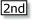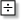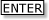Tapping into mathematics

Start this free course now. Just create an account and sign in. Enrol and complete the course for a free statement of participation or digital badge if available.

Free course

# 6.2 Getting the feel of big and small numbers

Very small and very large numbers can be difficult to comprehend. Nothing in our everyday experience helps us to get a good feel for them. For example numbers such as 1099 are so big that if Figure 1 was drawn to scale, you would be dealing with enormous distances. How big is big?

First express 1 000 000 000 in scientific notation as 109. Next, to find out how many times bigger 1099 is, use your calculator to divide 1099 by 109. This involves inputting the numbers in scientific notation. You may need to consult your calculator instructions on how to do this. On the TI-84, there is a button with the second function EE (this key has a comma , on it). You can enter the calculation on the TI-84 in two ways:[EE] 99[EE] 9or

1[EE] 991[EE] 9The second is entered strictly in scientific notation, as a number greater than or equal to 1 but less than 10, times a power of 10, e.g. 1 × 1099 or 1 × 109.

Enter the calculation in one or both of these forms, on your calculator, and you should get the answer equivalent to 1e90 or 1 × 1090. So if 109 is represented by 3 cm on the diagram, then to the same scale, 1099 should be represented by 3 × 1090cm. How far is that?

There are 100 cm in a metre and 1000 m in a kilometre. So there are 1 00 000 cm in a kilometre. This is 105 cm. So to convert distances in centimetres into kilometres you need to divide by 105. Using the same calculator procedure as before, divide 3 × 1090 by 105, to convert the distance from centimetres into kilometres.

You should get 3 × 1085, but 3 × 1085 km is further than the most distant galaxy in our universe. These are truly BIG numbers.

The next exercise gives you some calculator practice with big numbers.

## Exercise 12: Pieces of gold

Imagine that in exchange for this magic calculating device that you are holding in your hand, Queen Calcula of Sumwhere offers you either:

• (a) as many gold pieces as the number of minutes you have been alive;

or

• (b) as many gold pieces as the largest number you can get on your calculator using just five key presses;

or

• (c) one gold piece on the first day of this month, two on the second, four on the third, eight on the fourth, and so on ending on the last day of the month.

Which should you accept and why?

• (a) The number of minutes in a year is 60 × 24 × 365 (ignoring leap years).

• This is 525 600 or about half a million. So an eighty-year-old would receive about 40 million gold pieces. Not bad! 40 million is 4 × 107.

• (b) Probably the largest number on the calculator that can be obtained with five key presses is 1099. To get this number you press[EE] 9 9.

• (c) Let us assume that the month has 31 days.

 Day Gold on that day Total so far 1 2 3 4 5 ... 30 31 1 2 4=22 8=23 16=24 ... 229 230 1 3 7 15 31 ... ? ?
• The numbers in the third row of the table are one less than a power of 2:

 Total so far 1 3 7 15 31 ... ? ? =2−1 =4−1 =8−1 =16−1 =32−1 ... =21−1 =22−1 =23−1 =24−1 =25−1 ... =230−1 =231−1

The figure you want is the total so far in the final box (corresponding to day 31). Entering 231 − 1 on the calculator gives 2 147 483 647, which is the same as 2.147 483 647 ×109.

So part (b) produces far and away the largest number, 1099. However, you might be wondering whether it would be possible to deal with that many gold pieces. Is it possible to imagine a pile of that many coins? In fact, scientists have estimated that the total number of particles in the whole universe is only about 1080.

Well the exercise did start off with the word ‘imagine’!

MU120_4MCAL